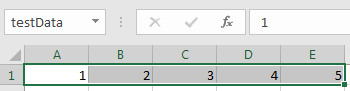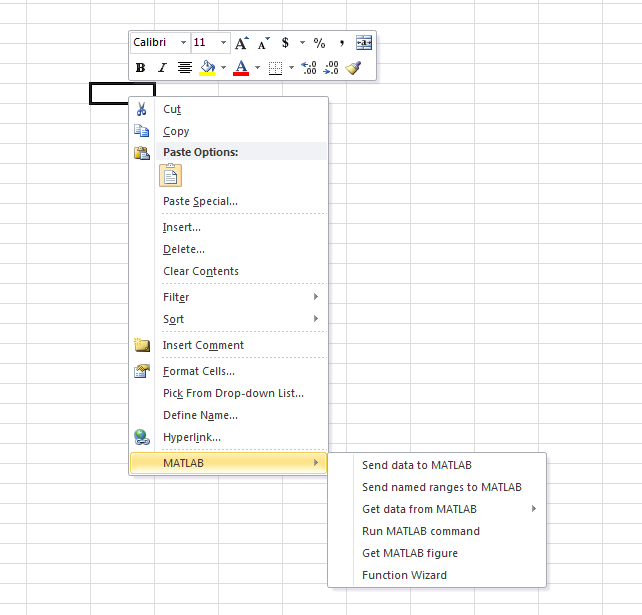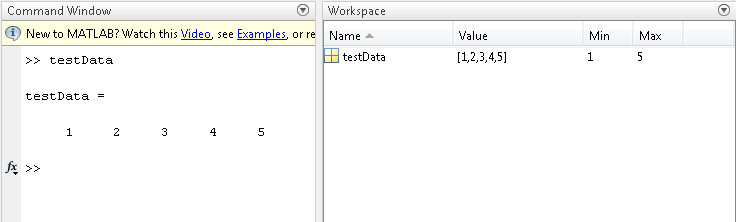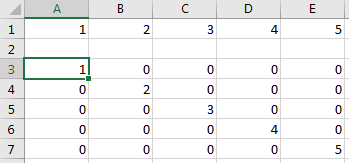## Create Diagonal Matrix Using Microsoft Excel Context Menu

This example shows how to execute Spreadsheet Link™ functions to export a named range in a worksheet to MATLAB® and create a diagonal matrix using the Microsoft® Excel® context menu.

The MATLAB group menu in the Microsoft Excel context menu contains commands for common Spreadsheet Link functions. For the list of common functions, see Execute Spreadsheet Link Functions.

This example assumes that MATLAB is running after Microsoft Excel opens. For details, see Start and Stop Spreadsheet Link and MATLAB.

In a worksheet, enter the numbers 1 through 5 into the range of cells from `A1` through `E1`. Define the name `testData` for this range of cells and select it. For instructions, see Excel Help and enter the search term: define and use names in formulas.

The named range `testData` appears in the Name Box.To find the command that exports the named range to MATLAB, right-click another cell outside of the named range in the worksheet. The Microsoft Excel context menu appears. To see the MATLAB group menu, select MATLAB.Select Send named ranges to MATLAB. When you select this command, the software executes `MLPutRanges`.

Microsoft Excel exports the named range `testData` into the MATLAB variable `testData` in the MATLAB workspace.Select the MATLAB group option Run MATLAB Command. When you select this command, Microsoft Excel displays a dialog box. Next, create a diagonal matrix. Use the `diag` function to specify `testData` as the input argument and `d` as the output argument. Enter this MATLAB command in the dialog box and click .

`d = diag(testData)`

The software executes the `MLEvalString` function. The MATLAB variable `d` appears in the MATLAB workspace and contains a diagonal matrix.

Retrieve the diagonal matrix into the worksheet. First select cell `A3`, and then select the MATLAB group option Get data from MATLAB > d. The software executes the `MLGetMatrix` function.

The diagonal matrix displays in cells `A3` through `E7`.